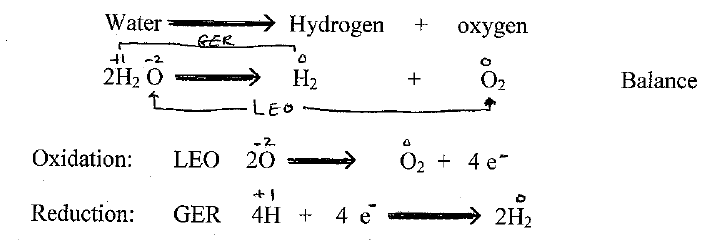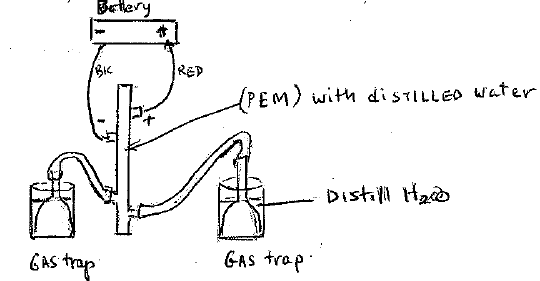How is a REDOX Reaction Used to Power a Car?

Palm Ng

Bayside High School, Bayside, Queens

Summer Research Program for Science Teachers

August 2008

These two lessons (45minutes) could be used after students have been introduced and have some understanding of  Reduction – Oxidation reactions (in the Electrochemistry Unit) to demonstrate a modern day application of Redox reaction.  If there is not enough time, then use the first lesson and just teacher demo the second (Can the Car Run on Hydrogen?).

Aim: How does the Hydrogen Fuel Cell produce energy to power a car?

(AKA: How is a  REDOX reaction used to power a car?)

Objectives:

SWBAT  1) Identify and label a Redox reaction

2) write a chemical equation using Redox

3) define Electrolysis

4) explain the electrolysis of water using Proton Exchange Membrane(PEM)

5) explain how a fuel cell works to power the model car

Motivation: Hydrogen Fuel Cell cars use hydrogen gas as fuel to power the car.

Where can we find an inexpensive source of hydrogen gas?    (water)

How can we get hydrogen gas from water?    (break it apart, process: electrolysis)

Development:

Q1)  What is electrolysis?

Electrolysis – The process in which electrical energy is used to bring a chemical change

Electro-lysis    (use electricity to lysis  (cut) )Demo/let students figure out (in small groups of 2 or 4s).

Q2)   What is happening? Write their answers.Materials:

Battery Pack

Silicon  tubing (2 pieces 1 1/2”)

(2 pieces 8’)

Containers (2)

Gas trappers(2)

Distilled water

Syringe,  Wires

PEM reversible fuel cell

Have student explain/share their observation and answers

How does the Hydrogen Fuel cell use Hydrogen gas to give energy to run the car?

Q3) How can we get energy from Hydrogen gas?  (moving electrons)

Q4) What kind of energy is it? (electricity- Flow of electrons. )

Q5) If we can get a flow of electrons(electricity) that would be the energy to run the car.

Q6) How can we get a flow of electrons from Hydrogen?  (elicit answers )

Explain the Proton Exchange Membrane (PEM) polymer Electrolyte membrane.

See attached diagram (PDF)From the PEM we get a flow of electrons (ELECTRICITY)

And the electricity is used to run/power the car.

Q7) What comes out the exhaust of the car?    ( Water)

Q8) Does water pollute?

HW: Read “ Honda Sees a Hydrogen Future ” article (PDF)

Write out the two chemical reactions needed to power a car.

Compare their similarities and differences (give two for each).

Tomorrow we will put the car(kit) together and see if  water can power the car.

NYSPS (New York State Performance Standards)

S1c – Demonstrates an understanding of chemical reactions

S5c – Uses evidence from reliable sources to develop description,      explanations and models; and makes appropriate adjustments     and improvements

S5e – Identifies problems; proposes and implements solutions; and     evaluates the accuracy, design, and outcomes of investigation

S5f – Works individually an in teams to collect and share information and ideas

S8c – Demonstrates scientific competence by completing a design